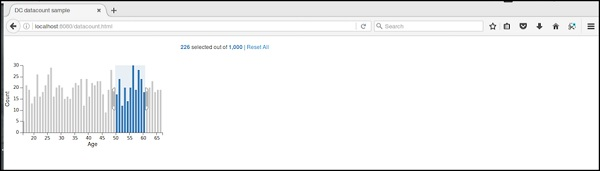# DC.js - Data Count

Data count is used to display the total number of records in the data set. It performs the following two types of count −

• Total-count − total number of records.

• Filter-count − number of records matched by the current filters.

## Data Count Methods

Before moving on to use a data count, we should understand the dc.dataCount class and its methods. The dc.dataCount class uses a mixin to get the basic functionality of displaying a data count, which is −

• dc.baseMixin

The dc.dataCount gets all the methods of this mixin and has its own method to show the data count as explained below −

### formatNumber( [formatter])

This method is used to get or set a format for the filter count and the total count.

### html( [options])

It is used get or set the HTML templates to show the number of selected items.

For example

```counter.html ({
all: 'HTML template to use if all items are selected'
})
```

Here, ‘all’ is used to select all the items using the %total-count. If we want to only use some of the items, then we can use some records using %filter-count option.

### Data count example

Let us perform the data count in DC. To do this, we need to follow the steps given below −

Let us add styles in the CSS using the coding given below −

```.dc-chart { font-size: 12px; }
```

Here, we have assigned styles for the chart.

### Step 2: Create a variable

Let us create a variable in DC as shown below −

```var barChart = dc.barChart('#line');
var countChart = dc.dataCount("#mystats");
```

Here, we have assigned a barChart variable id in line, whereas the countChart id is mystats.

### Step 3: Read the data

Read the data from the people.csv file as shown below −

```d3.csv("data/people.csv", function(errors, people) {
var mycrossfilter = crossfilter(people);
}
```

If the data is not present, then it returns an error. Now, assign the data to a crossfilter.

Here, we are using the people.csv file, which was used in our previous charting examples. It looks as shown below −

```id,name,gender,DOB,MaritalStatus,CreditCardType
1,Damaris,Female,1973-02-18,false,visa-electron
2,Barbe,Female,1969-04-10,true,americanexpress
3,Belia,Female,1960-04-16,false,maestro
4,Leoline,Female,1995-01-19,true,bankcard
5,Valentine,Female,1992-04-16,false,
6,Rosanne,Female,1985-01-05,true,bankcard
7,Shalna,Female,1956-11-01,false,jcb
8,Mordy,Male,1990-03-27,true,china-unionpay

.........................................
........................................
```

### Step 4: Set the dimension

You can set the dimension using the coding given below −

```// age dimension
var ageDimension = mycrossfilter.dimension(function(data) {
return ~~((Date.now() - new Date(data.DOB)) / (31557600000))
});
```

After the dimension has been assigned, group the age using the coding given below −

```var ageGroup = ageDimension.group().reduceCount();
```

### Step 5: Generate a chart

Now, generate a bar chart using the coding given below −

```barChart
.width(400)
.height(200)
.x(d3.scale.linear().domain([15,70]))
.yAxisLabel("Count")
.xAxisLabel("Age")
.elasticY(true)
.elasticX(true)
.dimension(ageDimension)
.group(ageGroup);
```

Here,

• We have assigned the chart width as 400 and height as 200.
• Next, we have specified the domain range as [15,70].
• We have set the x-axis label as age and y-axis label as count.
• We have specified the elasticY and X function as true.

### Step 6: Create and render the count chart

Now, create and render the count chart using the coding below −

```countChart
.dimension(mycrossfilter)
.group(mycrossfilter.groupAll());

barChart.render();
countChart.render();
```

Here, we have assigned the dimension to a crossfilter variable. Finally, group all the records based on the age.

### Step 7: Working example

The complete code is as follows. Create a web page datacount.html and add the following changes to it.

```<html>
<title>DC datacount sample</title>
<link rel = "stylesheet" type = "text/css" href = "css/bootstrap.css" />
<link rel = "stylesheet" type = "text/css" href = "css/dc.css" />

<style>
.dc-chart { font-size: 12px; }
</style>

<script src = "js/d3.js"></script>
<script src = "js/crossfilter.js"></script>
<script src = "js/dc.js"></script>

<body>
<div>
<div style = "width: 600px;">
<div id = "mystats" class = "dc-data-count" style = "float: right">
<span class = "filter-count"></span> selected out of <span
class = "total-count"></span> | <a href = "javascript:dc.filterAll();
dc.renderAll();">Reset All</a>
</div>
</div>

<div style = "clear: both; padding-top: 20px;">
<div>
<div id = "line"></div>
</div>
</div>
</div>

<script language = "javascript">
var barChart = dc.barChart('#line'); // , 'myChartGroup');
var countChart = dc.dataCount("#mystats");

d3.csv("data/people.csv", function(errors, people) {
var mycrossfilter = crossfilter(people);

// age dimension
var ageDimension = mycrossfilter.dimension(function(data) {
return ~~((Date.now() - new Date(data.DOB)) / (31557600000))
});
var ageGroup = ageDimension.group().reduceCount();

barChart
.width(400)
.height(200)
.x(d3.scale.linear().domain([15,70]))
.yAxisLabel("Count")
.xAxisLabel("Age")
.elasticY(true)
.elasticX(true)
.dimension(ageDimension)
.group(ageGroup);

countChart
.dimension(mycrossfilter)
.group(mycrossfilter.groupAll());

barChart.render();
countChart.render();
});
</script>
</body>
</html>
```

Now, request the browser and we will see the following response.

Data count initial page is shown below.

After selecting a particular age, it shows the counts as shown in the screenshot below.